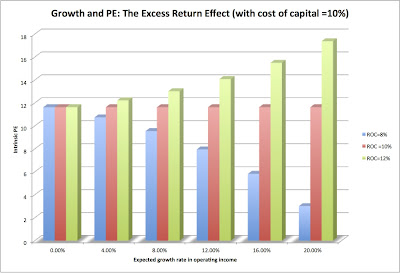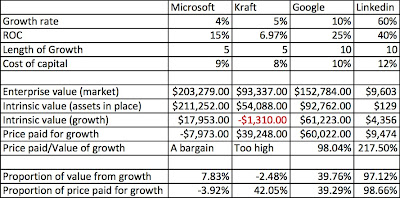By

In my last post, I looked at the price being paid for growth by valuing the assets in place in a business. To make this judgment, I assumed that the business would pay its entire operating income to claim holders (as dividends to stockholders and interest expenses to lenders). The value of assets in place then becomes the value of the earnings in perpetuity, discounted back at the cost of capital.

So, what is the effect of growth on value? To grow in the long term, you have to reinvest some or a big portion of your earnings back into the business, and the amount you have to reinvest will depend upon the return on capital you earn on your new investments:

Reinvestment Rate = Expected Growth rate/ Return on Capital

Thus, a firm with a return on capital of 15% that wants to grow 3% a year will have to reinvest 20% (= 3%/15%) of its operating income back each year. Investors will thus get less in cash flows up front but have higher cash flows in future years.

Consider an example. A firm that generates \$ 10 million in after-tax operating income, and has a cost of capital of 10%, will have a value of assets in place of \$100 million, if it pursues a "no growth" policy:

Value of assets in place = \$10 million/ .10 = \$100 million

If it decides to pursue a 3% growth rate and invest 20% of its after-tax income (based upon the return on capital of 15%), its value can be computed as follows:

Value of firm = 10 million (1-.20) / (.10-.03) = \$114.28 million

The difference between the two values then becomes the value added by growth:

Value of growth = Value of firm – Value of assets in place = \$114.28 - \$100 = \$14.28 million

Determinants of the value of growth

If you accept the proposition that growth creates a trade off of lower cash flows today for higher ones in the future, you have the three ingredients that determine the value of growth. The first is the level of growth, with higher growth rates in the future generating higher earnings over time. The second is how long these high growth rates can be sustained before the company becomes too big to keep growing (at least at rates higher than that of the economy). The third and most critical is the return on capital you generate on new investments.

To see why the last ingredient is so critical, revisit the last example and make the return on capital = cost of capital. If you do so, the reinvestment rate has to be 30% to sustain the expected growth rate of 3%. The value of growth then becomes zero:

Value of firm = 10 million (1-.30) / (.10-.03) = \$100 million

Value of growth = Value of firm – Value of assets in place = \$100 - \$100 = \$ 0

In fact, if the return on capital generated on new investments is less than the cost of capital, growth can destroy value.

The process of valuing growth does get a little more complicated when you set higher growth rates, but the logic and conclusions do not change. If the return on capital > cost of capital, the value of growth will increase as the growth rate increases and the length of the growth period expands. If the return on capital = cost of capital, neither the growth rate nor the length of the growth period affect value and if the return on capital < cost of capital, the value will move inversely with the growth rate and the length of the growth period. If you want to take this concept out for a trial run, this spreadsheet can help you.

Comparing the value of growth to the price paid for growth

If you are paying a price for growth, it is always useful to know the value of this growth. If you accept the reasoning in the last section, it follows that it is not growth that you should be paying a premium for but “quality growth”, with quality defined as the excess return you generate over and above the cost of capital. To illustrate this concept, we compute “intrinsic” PE ratios at varying growth rates for three firms, all of which share a cost of capital of 10% but vary in the returns on capital that they earn on new investments (one has a return on capital of 8%, the second has a return on capital of 10% and the third has a return on capital of 12%).The PE ratio for just the assets in place is 11.63 and remains unchanged, even if you introduce growth, for a firm that earns its cost of capital. For the firm that generates a return on capital cost of capital, the PE ratio does increase (the growth premium) as growth increases. It is this premium that you would compare to what you actually pay to make a judgment on whether the added PE you are paying for growth is justified.

Price of Growth versus Value of Growth

Using the spreadsheet on growth as a device for deconstructing growth (and its value), I looked at Microsoft, Kraft, Google and Linkedin. In the table below, I have listed my base assumptions for each company and the value of assets in place and expected growth in each one:This table can be used to address several issues relating to growth:

a. Price of growth versus value of growth: You can compare the price you are paying for growth with the value of growth, and you come to different conclusions. For Microsoft, where the value of assets in place covers the market price you are paying, the value of growth is a pure bonus. For Kraft, the value of growth is negative, since the firm earns less than its cost of capital, and the price you are paying for growth is therefore too high. For Google, the price of growth is almost exactly equal to the value of growth, making it the only fair priced stock in this grouping. Finally, for Linkedin, the price paid for growth is more than twice the value of that growth, making the stock over valued. For investors who believe in growth at a reasonable price (GARP), this is the statistic worth watching.

b. Implied growth rates: An alternative approach is to solve for that growth rate (Look at the spreadsheet and follow the instructions), holding the return on capital and length of growth period fixed, that would yield the price you paid for that growth. Linkedin, for instance, would have to maintain a compounded growth rate of 73% a year (instead of the estimated growth rate of 60% a year) for the next ten years to justify the price you are paying for the growth. (The spreadsheet provides instructions on how to back out the implied growth rate using the Goal seek function in Excel.)

Growth, in summary, does not yield itself easily to rules of thumb or broad generalizations. In some firms, it can be worth nothing, as is argued by strict value investors, whereas in others, it can be worth a great deal, lending credence to the arguments of growth investors.

(Aswath Damodaran is a professor of finance at the Stern School of Business at NYU.)